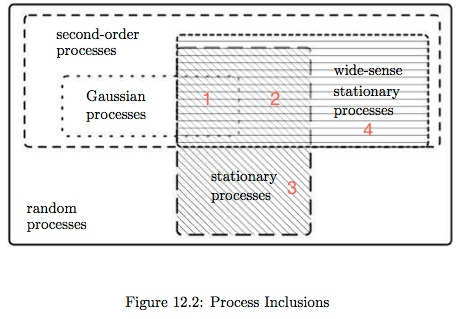Probability is interchangeable with proportion; although one is theoretical, the other arithmetical.

## Axiomatic Probability

• Probability space;
• Conditional probability;
• Total probability equation, Bayes formular;
• Independence;

## Random Variable

• Measurable function on probability space;
• Distribution: CDF, PDF, PMF;
• Integration (Expectation), Riemann-Stieltjes integral;
• Characteristic function and moments
• Function Analytic Approach to Probability;
• Reference Distribution;
• Distributions from Discrete Random Process;
• Distributions from Continuous Random Process;
• Asymptotic Distributions;
• Gaussian-related Distributions;
• Conditional expectation;
• Hierarchical models;
• Independence as an assumption and simplification;
• Covariance and correlation;

Special topics:

## Random Process

Note that mathematical theories go complicated very fast. In fact, the description of a random process already requires too much information, which is impractical. To put the mathematical model into practical use, vast simplification is needed.

Stochastic analysis:(Ref: Scholtz, P266.)

Random processes:

Nassim Nicholas Taleb, Skin in the game:

"Ergodicity is not statistically identifiable, not observable, and there is no test for time series that gives ergodicity, similar to Dickey-Fuller for stationarity (or Phillips-Perron for integration order)." "If your result is obtained from the observation of a time series, how can you make claims about the ensemble probability measure?"

## Misc

Fourier Transforms, Z-transform:

Table: Fourier Transform and Its Inverse Transform as the Integral Transforms Defined by Kernels. (In the form of $a e^{bixy}$, where $a$ is normalization and $b$ is oscillatory factor.)

Convention Forward kernel Inverse kernel
physics $e^{-ixy}$ $(2\pi)^{-1}e^{ixy}$
physics, symmetric $(2\pi)^{-1/2} e^{-ixy}$ $(2\pi)^{-1/2} e^{ixy}$
mathematics $e^{-2\pi ixy}$ $e^{2\pi ixy}$
general $│b│^{1/2}(2\pi)^{(-1+a)/2}e^{-ibxy}$ $│b│^{1/2}(2\pi)^{(-1-a)/2}e^{ibxy}$

Sinc function $\text{sinc}(x) = \frac{\sin x}{x}$, $x \ne 0$. Normalized sinc function $\text{sinc}(x) = \frac{\sin(\pi x)}{\pi x}$, $x \ne 0$, because its integral on the real line equals one: $\int_{-\infty}^\infty \frac{\sin(\pi x)}{\pi x} = 1$. Rectangular function is the centered unit-length indicator function: $\text{rect}(x) = 1(|x| \le 1/2)$. The Fourier transform (in math convention) of the normalized sinc / rectagular function is the rectagular / normalized sinc function. The Fourier transform (in physics convention) of the sinc function is the constant function that equals pi in between -1 and 1: $\int_{-\infty}^\infty e^{-ixt} \frac{\sin x}{x} = \pi \cdot 1(|t| \le 1)$.

Table: Interpretations of probability

Ontic (实存) probability Epistemic (认识) probability
long run frequency objective degree of belief
single-case propensity subjective degree of belief